You can add a multiplicative overdispersion parameter to a generalized linear model in the GLIMMIX procedure with the statement

``` random _residual_;
```

For models in which, this effectively lifts the constraint of the parameter. In models that already contain a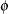orscale parameter—such as the normal, gamma, or negative binomial model—the statement adds a multiplicative scalar (the overdispersion parameter,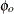) to the variance function.

The overdispersion parameter is estimated from Pearson’s statistic after all other parameters have been determined by (restricted) maximum likelihood or quasi-likelihood. This estimate is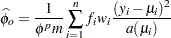where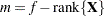if the NOREML option is in effect, and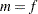otherwise, and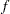is the sum of the frequencies. The powerisfor the gamma distribution andotherwise.

Adding an overdispersion parameter does not alter any of the other parameter estimates. It only changes the variance-covariance matrix of the estimates by a certain factor. If overdispersion arises from correlations among the observations, then you should investigate more complex random-effects structures.